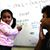D

#### I am getting different answers for a Physics problem

91 viewed last edited 4 years agoAnonymous
2
I am confused about how to solve the following problem: A ball is rolled up a frictionless inclined plane making an angle of 30 degrees with the ground. If the ball's velocity is 1m/s up the plane, how high does the ball get above the ground? I am getting two answers: First method: The component of velocity of the ball along the vertical axis is 0.5 m/s. Since the acceleration due to gravity is 9.8 m/s^2 pointing downwards, the maximum height is given by formula v^2 = u^2 - 2gh. From there I get, h = 0.5*0.5/(2*9.8) m Second method: The loss in kinetic energy should be equal to the gain in potential energy. Therefore, 1/2 m 1 * 1 = m g h. From there I get, h = 1/(2*9.8) m. I know the second method is correct and that should be my answer. However, what am I doing wrong in the first one?S. Prem Kumar
4

The first method is incorrect because if you break up the velocity components into vertical and horizontal then you need to take into account all vertical (and horizontal) forces. The vertical forces include gravity and a component of the normal reaction from the inclined plane on the ball. You did not include the latter.

You are much better off looking at the forces along and perpendicular to the inclined plane and applying the acceleration formula along the plane. Along the plane there is only one force acting to decelerate the ball, which is the component of mg along the plane. This calculation is actually identical to the energy argument.Mahesh Godavarti
2

Let me see if I can add more details to Prem's answer. Consider the diagram below. There are three vectors in play here. Acceleration due to gravity in black, the normal force acting on the ball in blue and the ball's velocity in brown.

I have broken the vectors into its components based in each direction.There are two ways to solve this. The first is to consider directions parallel and perpendicular to direction of gravity (we will call them vertical and horizontal directions), and the other is to consider directions parallel and perpendicular to the plane.

APPROACH 1: Let's take the first approach first (that is what you were considering). You broke the velocity into two components, horizontal and vertial. Note that acceleration experienced by the ball in the vertical direction includes both the acceleration due to gravity as well as the component of acceleration from the normal force acting on the ball (in blue). The normal force = component of the ball's weight perpendicular to the plane = m g \sin 30^\circ = \frac{\sqrt{3}}{2} m g . Now the total acceleration in the vertical direction is given by

= g - component of normal acceleration in the vertical direction

= g - \frac{\sqrt{3}}{2} \frac{\sqrt{3}}{2} g = \frac{1}{4} g .

The component of velocity in the vertical direction = 1 \cos 30^\circ = \frac{1}{2} m/s.

Therefore, total distance traveled in the vertical direction = \frac{(0.5)^2}{2 \frac{1}{4} g} = \frac{1}{2g} . This is the same as what you would get with the conservation of energy. Wow!

APPROACH 2: Let's consider the directions perpendicular and parallel to the plane. The acceleration the ball experiences along the plane is \frac{1}{2} g . Therefore, total distance covered along the plane = \frac{1}{2 \frac{1}{2} g} = \frac{1}{g} . Note that this is the distance covered along the plane. The vertical distance covered is half of this giving you \frac{1}{2g} . Exactly the same approach as APPROACH 1.

Of course, the final approach is to use the conversation of energy principle which gives you the same answer.

Hope this helps.